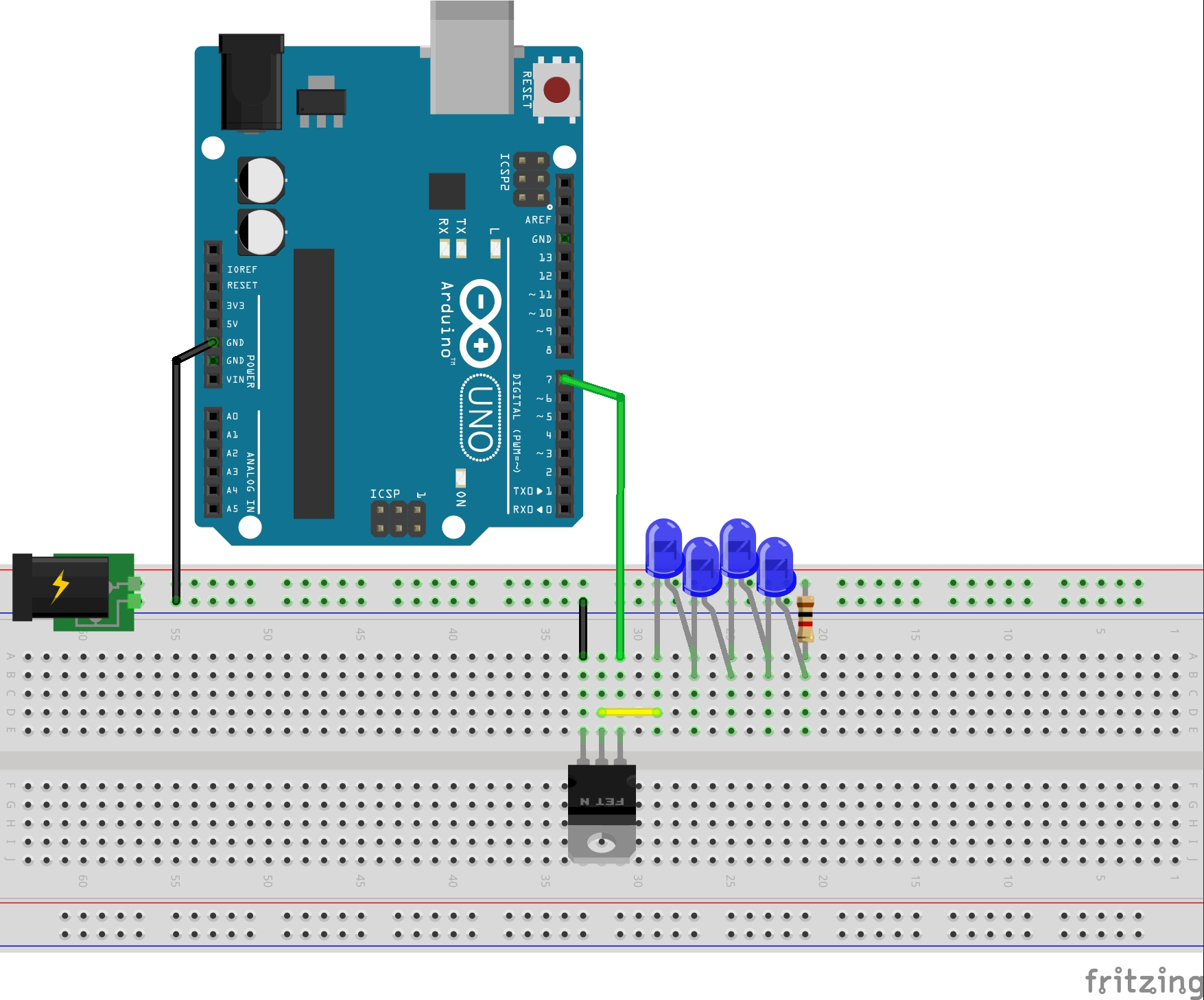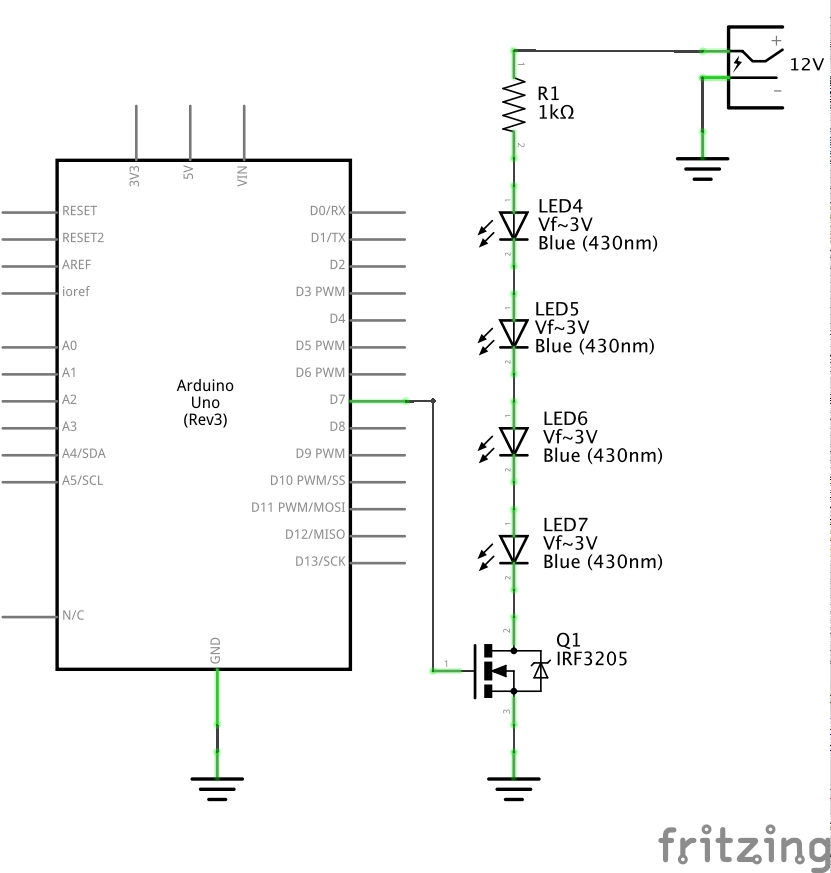#286 SwitchPowerNFET

Low-side n-channel MOSFET switching of large loads with a microcontroller.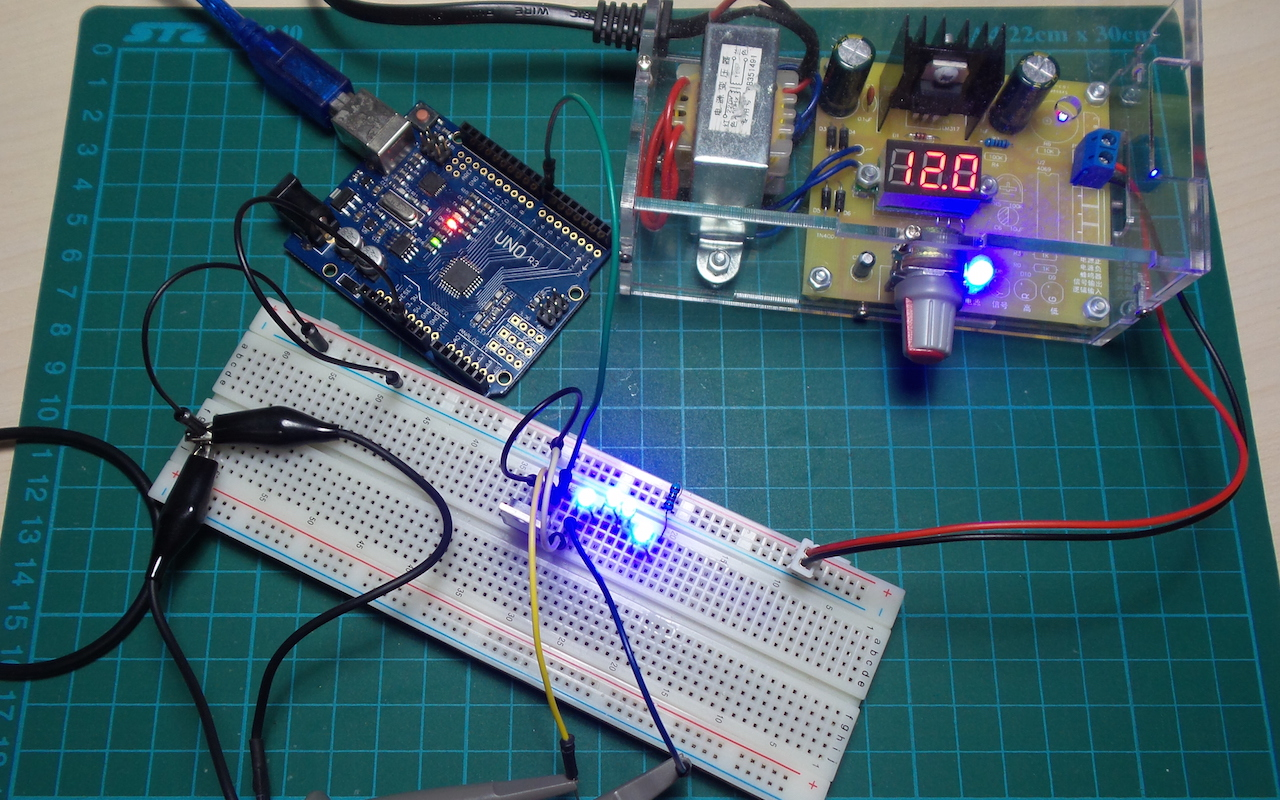The LEAP#66 SwitchNFET project demonstrated basic n-channel enhancement mode switching.

This is a common variation, where the gate control (switching) voltage is much lower than the voltage across the switched load. For example, using a 5V Arduino to switch a 24V load.

The standard configuration in such cases is low-side switch - where the MOSFET source is at the same voltage as the switching circuit ground.

The switching circuit will then toggle the MOSFET gate voltage between its high and low levels (say 0V and 5V for a 5V Arduino). But the voltage on the load side can be much higher.

The primary consideration is to ensure that the switching circuit voltage is large enough to turn the MOSFET hard on. This depends on the particular FET gate threshold voltage (Vgs) which will be listed in the datasheet.

In this example, I’m using an IRF3205 n-FET which has a Vgs of 2.0 to 4.0V. So a 5V switch circuit should be able to drive it hard on and off without any trouble.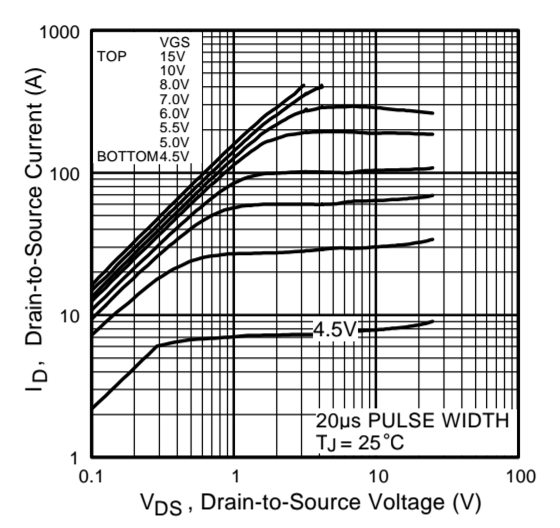Demonstration

As an example load, I am simply switching a series of LEDs with a 12V power supply.

The SwitchPowerNFET.ino sketch simple toggles the gate voltage every 5ms (i.e. at 100Hz). Since I’m using an Arduino Uno, the gate voltage will switch between 0V and 5V.

Performance

The first scope trace compares Vgs and Vds. With a LED load we get a little bit of a “soft-landing” when switching off.

• CH1 (yellow): Vgs, offset -6V
• CH2 (blue): Vds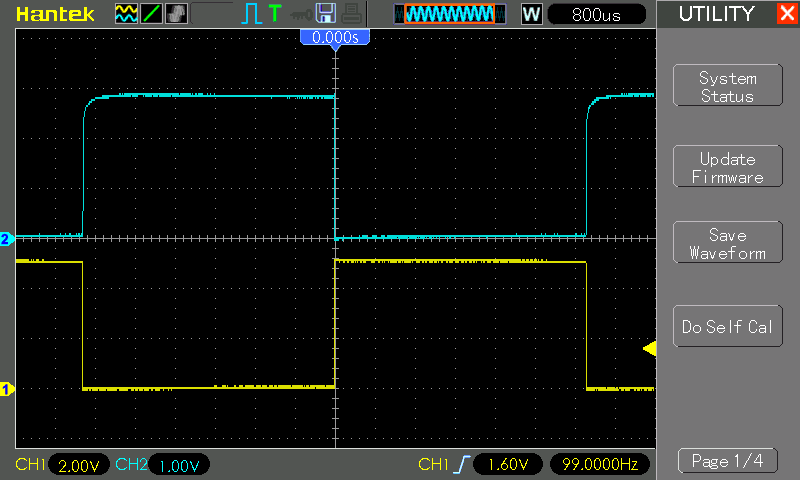To measure the current and to verify hard switching, this trace shows the voltage across the resistor:

• when off, Vr = 0V
• when on, Vr is approximately 1.4V, for a current of 1.4mA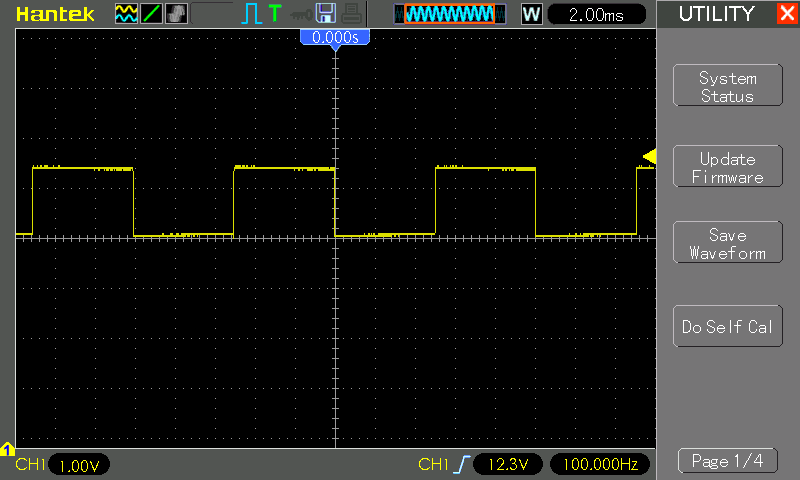With a pure 10kΩ resistive load, it’s easier to see the hard on/off control.

• CH1 (yellow): Vgs, offset -6V
• CH2 (blue): VdsConstruction